Angular Quantities

Consider a small particle moving in a circle with radius "r" along the circumference from point A to point B tracing an arc length "s"
.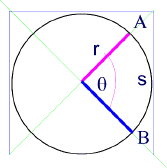When the arc s = r ,  Ө = 1 radian measure, therefore Ө = s / r

Wher the angle Ө is measured in radians.

•  Ө is known as the angular distance.
•  s  is the linear distance (generally called  "d")

d  =  r  Ө

Since Ө = s / r (or Ө = d / r), we can relate the linear distance d to the angular distance  Ө using the simple equation:

Recall that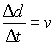This can be obtained by dividing the left side of the above equation by ∆t).

Dividing the right side of the same equation by ∆t we obtain the following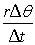.

The factor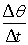is known as the angular velocity.
The symbol for angular velocity is ω (pronounced "omega").  Its units are "radians per second -- rad/s".

Where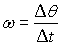andWe can also express the angular velocity ω in terms of the frequency of rotation "f".

Starting from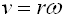we get...Therefore:  ω = 2πf

In this case f is measured in revolutions per second (Hz) and ω is measured in radians per second

##### Similarly we can show that by dividing both sides of the equation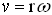bywe can obtain expressions for acceleration.• The left side of the equation defines the linear acceleration "a".
• The right side of equation defines the angular acceleration "a(pronounced "alpha").

Recall that  in a full circle, the total radian/angular measure is  θ = 360

The linear distace around the circle, known as circumference, is d = 2 π r (the total circumference of the circle).

We measure θ in radians (a linear measure) instead of degrees.

If d = r θ, then  2πr =  r or simply  2π [radians] = 3600 ,

Consequently the units of the acceleartion, a are m/s2  and the  units of "a" are radians/s2  [rads/s2

##### Comparing Angular equations of Motion to Linear Quantities
 Linear Equations of Motion Angular Equations of Motion v2 = v1 + a t ω2= ω1 + a t v22  = v12 + 2 a d ω22= ω12 + 2aӨ d = v1t + 1/2 at2 Ө = ω1 t+ 1/2at2 vav = (v1 + v2) / 2 ωav = (ω1 + ω2 ) / 2 d  =  r Ө ω = 2 π f